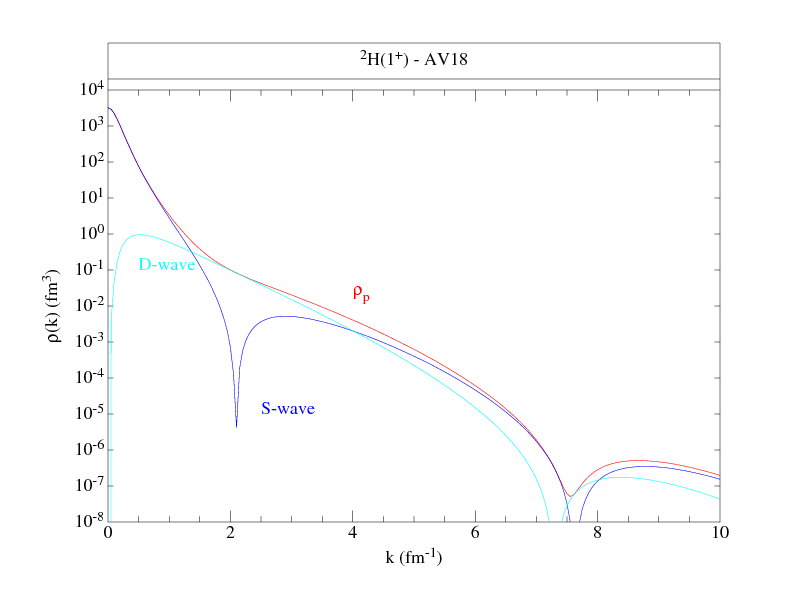# Single-Nucleon Momentum Distributions

## Two-Nucleon Momentum DistributionsThis web page presents single-nucleon momentum distributions calculated for a variety of nuclei in the range A=2-12 as discussed in Wiringa, Schiavilla, Pieper, and Carlson: Phys. Rev. C 89, 024305 (2014) (or arXiv:1309.3794). Corresponding two-nucleon momentum distributions can be found here. These are from variational Monte Carlo calculations (VMC) using (unless otherwise noted) the Argonne v18 two-nucleon and Urbana X three-nucleon potentials (AV18+UX). (Urbana X is intermediate between the Urbana IX and Illinois-7 models; it has the form of UIX supplemented with a two-pion S-wave piece, while the strengths of its terms are taken from the IL7 model. It does NOT have the three-pion-ring term of IL7.)

These VMC wave functions are the starting trial functions for a number of recent Green's function Monte Carlo (GFMC) calculations:
Brida, et al., Phys. Rev. C 84, 024319 (2011);
McCutchan, et al., Phys. Rev. C 86, 024315 (2012);
Pastore, et al., Phys. Rev. C 87, 035503 (2013);
Datar, et al., Phys. Rev. Lett. 111, 062502 (2013);
Pastore, et al., Phys. Rev. C 90, 024321 (2014).

More details of the wave function construction can be found in
Wiringa, Phys. Rev. C 43, 1585 (1991) for A=3,4;
Pudliner, et al., Phys. Rev. C 56, 1720 (1997) for A=6,7;
Wiringa, et al., Phys. Rev. C 62, 014001 (2000) for A=8;
Pieper, et al., Phys. Rev. C 70, 044310 (2002) for A=9,10.

The largest nuclei are evaluated using the cluster VMC (CVMC) method.

The CVMC method is described in
Pieper, et al., Phys. Rev. C 46, 1741 (1992) for A=16 with AV14+UVII
Lonardoni, et al., Phys. Rev. C 96, 024326 (2017) for A=16,40 with AV18+UIX.

The results are generated as distributions for neutron spin-down, neutron spin-up, proton spin-down, and proton spin-up, for the M=J state. The single-nucleon densities corresponding to these wave functions are given here

### Spin-polarized nucleon momentum distributions as functions of k

Following are files that tabulate the proton and neutron momentum distributions along with figures to give an overall view of the distributions. The normalization is chosen such that:

ANS = 1/(2π)3 ∫ d3k ρNS(k)

where NS denotes proton or neutron, spin up or down, and ANS is the total number (out of A) nucleons with the given nucleon-spin projection. Where proton and neutron momentum distributions are the same, as in T=0 nuclei, we give only one set, and similarly, if spin-up and spin-down projections are the same, as in 0+ states, we give totals only. The kinetic energy from these distributions is also given.

--- NEW! ---
Results for some of the Norfolk NV2+3 potentials are now being added:
Piarulli, et al., Phys. Rev. C 94, 054007 (2016).
--- NEW! ---

 2H(1+) AV18 Figure Table 2H(1+) NV2-Ia Figure Table 2H(1+) NV2-Ib Figure Table 2H(1+) NV2-IIa Figure Table 2H(1+) NV2-IIb Figure Table 3H(1/2+) Figure 1 Figure 2 Figure 3 Table Table (dn) 3He(1/2+) Figure 1 Figure 2 Figure 3 Table Table (dp) 4He(0+) Figure 1 Figure 2 Table Table (tp+dd) 4He(0+) AV18+UIX Figure Table 4He(0+) AV18 Figure Table 6He(0+) Figure 1 Figure 2 Table 6Li(1+) Figure 1 Figure 2 Figure 3 Table Table (αd) 6Li(3+) Figure 1 Figure 2 Figure 3 Table Table (αd) 7Li(3/2-) Figure 1 Figure 2 Figure 3 Table Table (αt) 7Li(1/2-) Figure 1 Figure 2 Figure 3 Table Table (αt) 7Li(7/2-) Figure 1 Figure 2 Figure 3 Table Table (αt) 7Li(5/2-) Figure 1 Figure 2 Figure 3 Table Table (αt) 8He(0+) Figure 1 Figure 2 Table 8Li(2+) Figure 1 Figure 2 Figure 3 Table 8Be(0+) Figure 1 Figure 2 Table Table (αα) 8Be(2+) Figure 1 Figure 2 Table Table (αα) 8Be(4+) Figure 1 Figure 2 Table Table (αα) 8B(2+) Figure 1 Figure 2 Figure 3 Table 9Li(3/2-) Figure 1 Figure 2 Table 9Be(3/2-) Figure 1 Figure 2 Table 10He(0+) Figure 1 Figure 2 Table 10Be(0+) Figure 1 Figure 2 Table 10B(3+) Figure 1 Figure 2 Table 11Li(3/2-) Figure 1 Figure 2 Table 11B(3/2-) Figure 1 Figure 2 Table 12Be(0+) Figure 1 Figure 2 Table 12C(0+) Figure Table 12C(0+) AV18+UIX Figure Table 16O(0+) CVMC AV18+UIX Figure Table 16O(0+) CVMC AV18 Figure Table 40Ca(0+) CVMC AV18+UIX Figure Table 40Ca(0+) CVMC AV18 Figure Table

Robert B. Wiringa
Last updated September 18, 2020